# 网络流：最小割

## S - T 最小割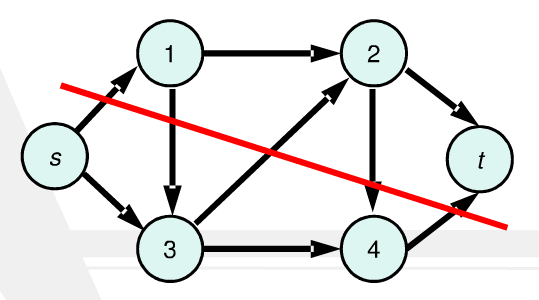## 最大流最小割定理

### 证明 Step 1：任意一个流都小于等于任意一个割

$$\forall F \leqslant \forall C$$

### Step 3：最大流等于最小割

$\therefore \forall F \leqslant F_m=C_m \leqslant \forall C$。

### 网络流等价定理

1. 流 $f$ 是图 $G$ 的最大流；

2. 残留网络 $G$ 不存在增广路；

3. 在 $G$ 中必存在一个割 $C[S,T]$，使得 $f=C[S,T]$。

## 最大权闭合子图

### 栗子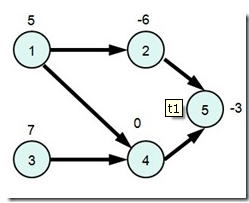## 最大权闭合子图权值和

### 构图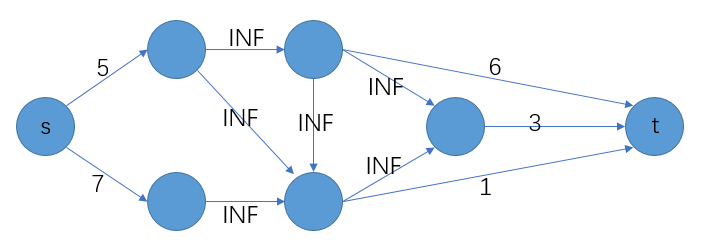（注：点权为 0 的点可以忽略，对结果没有影响）

### 前置知识

• 该带边权有向图的 S - T 最小割，割集中所有的边，都与 s 或 t 相连接。

• 该图中的每一个简单割产生的两个子图，我们记含有点 s 的是图 S，含有点 t 的是图 T，则图 S 是最大权闭合子图。

$$w+x=w_1-w_2+x_1-x_2$$

$$x_2 = w_2$$

$$w+x=w_1+x_1$$

$$w=sum-x$$

### 栗子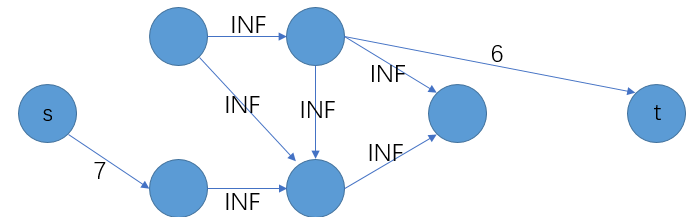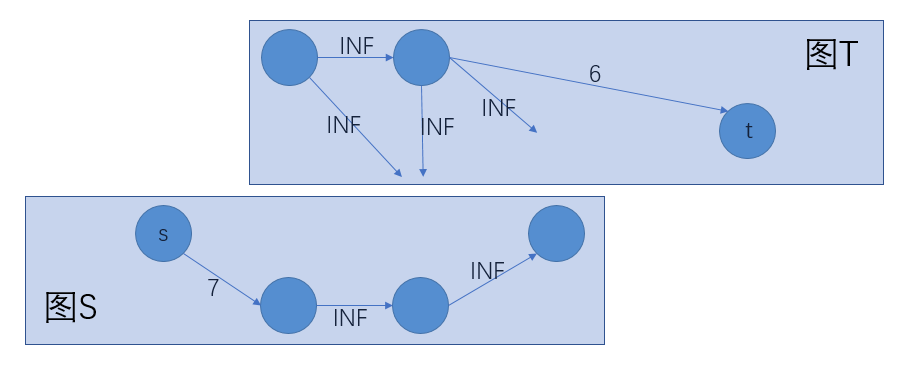### 解法

• 先记录整个图中，所有正点权值的和；

• 建立对应流网络，求最大流，最大流在数值上等于最小割，故我们得到了流网络的 s-t 最小割；

• 所有正点权值的和减去 s-t 最小割，即得最大权闭合子图的权值和。

2020-05-14

2023-01-11